# Newton's LawsPage 1

#### WATCH ALL SLIDES

Slide 1Newton's Laws

Slide 2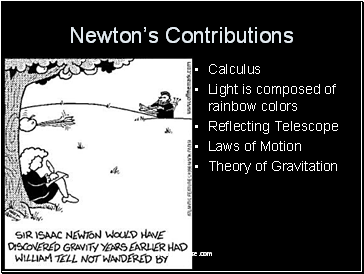## Newton’s Contributions

Calculus

Light is composed of rainbow colors

Reflecting Telescope

Laws of Motion

Theory of Gravitation

Slide 3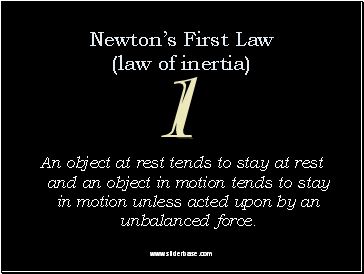Newton’s First Law (law of inertia)

An object at rest tends to stay at rest and an object in motion tends to stay in motion unless acted upon by an unbalanced force.

Slide 4## Balanced Force

Equal forces in opposite

directions produce no motion

Slide 5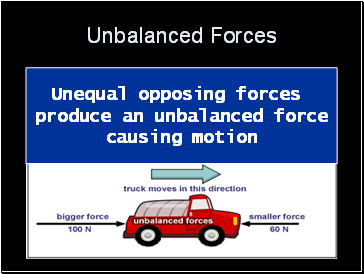## Unbalanced Forces

Unequal opposing forces

produce an unbalanced force

causing motion

Slide 6If objects in motion tend to stay in motion, why don’t moving objects keep moving forever?

Things don’t keep moving forever because there’s almost always an unbalanced force acting upon them.

A book sliding across a table slows down and stops because of the force of friction.

If you throw a ball upwards it will eventually slow down and fall because of the force of gravity.

Slide 7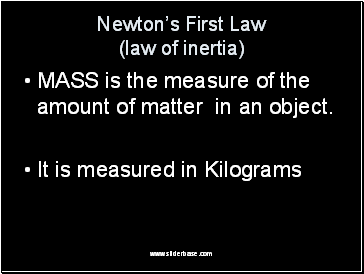## Newton’s First Law (law of inertia)

MASS is the measure of the amount of matter in an object.

It is measured in Kilograms

Slide 8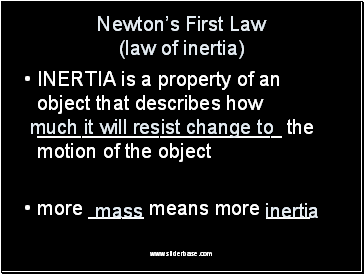Newton’s First Law (law of inertia)

INERTIA is a property of an object that describes how the motion of the object

more _ means more

much it will resist change to

mass

inertia

Slide 91st Law

Unless acted upon by an unbalanced force, this golf ball would sit on the tee forever.

Slide 10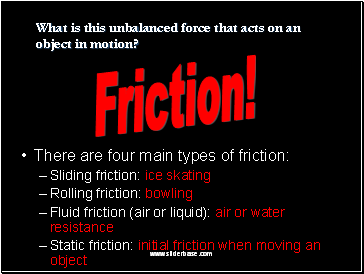There are four main types of friction:

Sliding friction: ice skating

Rolling friction: bowling

Fluid friction (air or liquid): air or water resistance

Static friction: initial friction when moving an object

Friction!

What is this unbalanced force that acts on an object in motion?

Slide 111st Law

Once airborne, unless acted on by an unbalanced force (gravity and air – fluid friction) it would never stop!

Slide 12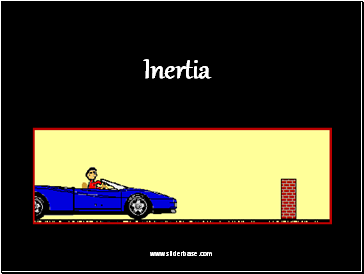Go to page:
1  2  3